Design of Three-Dimensional Missile Guidance Law via Sliding Mode with Saturation Constraint | OMICS International
Journal of Electrical & Electronic Systems

# Design of Three-Dimensional Missile Guidance Law via Sliding Mode with Saturation Constraint

Amir Torabi*, Amin Adine Ahari and Ali Karsaz

Faculty of Electrical Engineering, Khorasan University, Mashhad, Iran

*Corresponding Author:
Amir Torabi
Faculty of Electrical Engineering
E-mail: [email protected]

Received Date: March 30, 2013; Accepted Date: May 27, 2013; Published Date: May 29, 2013

Citation: Torabi A, Ahari AA, Karsaz A (2013) Design of Three-Dimensional Missile Guidance Law via Sliding Mode with Saturation Constraint. J Electr Electron Syst 2:107. doi:10.4172/2167-101X.1000107

Copyright: © 2013 Torabi A, et al. This is an open-access article distributed under the terms of the Creative Commons Attribution License, which permits unrestricted use, distribution, and reproduction in any medium, provided the original author and source are credited.

Visit for more related articles at Journal of Electrical & Electronic Systems

#### Abstract

The three-dimensional missile guidance law with saturating actuator is proposed for the pursuit of an unpredictably maneuvering target. The proposed guidance law achieves a satisfactorily robust performance without having to solve a complicated nonlinear Hamilton-Jacobi Partial Differential Inequality (HJPDI). In the proposed guidance scheme, the nonlinear part of the relative motion equations between the missile and target is first cancelled. Then, a tunable sliding mode law is designed to eliminate the effect of external disturbance. The proposed guidance law can achieve reduced interception time than others, yet it is considerably simpler. An example is proposed to confirm it.

#### Keywords

Missiles; Sliding mode law

#### Nomenclature

r- Relative distance between the missile and the target

F-Pitch line-of-sight angle (PLOS)

Θ-Yaw line-of-sight angle (YLOS)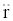-Relative acceleration along LOS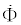-Angular velocity of F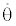-Angular velocity of Θ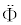-Angular acceleration of F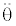-Angular acceleration of Θ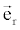-Unit vector along LOS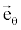-Unit vector along YLOS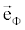-Unit vector along PLOS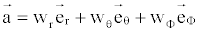-Acceleration vector of target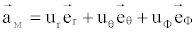-Acceleration vector of missile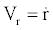-Relative velocity along to LOS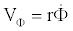-Relative velocity normal to PLOS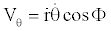-Relative velocity normal to YLOS

#### Introduction

The missile guidance is a well-known nonlinear control engineering area of research. Since the basic principles were extensively covered by Locke  and Lin , many technologies have been developed to improve guidance performance and to overcome environmental disturbances.

Various guidance laws have been exploited with different design concepts over the years . Currently, the most popular terminal guidance laws, as defined by Locke , involve Line-Of-Sight (LOS) guidance, LOS rate guidance and other advanced guidance such as proportional navigation guidance , Augmented Proportional Navigation Guidance (APNG) , optimal guidance law based on the linear quadratic regulator theory  and linear exponential Gaussian theory .

The variable structure control law has been applied to many guidance problems. For example, Zhou et al.  constructed the adaptive slidingmode guidance using linearised equations. Babu et al.  proposed the guidance law against highly maneuvering targets using the sliding surface of the zero LOS rate based on the Lyapunov method. Brierly and Longchamp  presented the sliding mode guidance law including rigid-body dynamics and actuator time delay. Moon et al.  studied the sliding-mode missile guidance law via variable structure control.

Recently, Chen et al.  derived a new nonlinear fuzzy H guidance law with a saturation of actuators against maneuvering targets. They first approximated the nonlinear missile target pursuit dynamics with the Takagi - Sugeno linear fuzzy models. Then, based on this, they constructed a H control to handle the approximation error and external disturbances. However, it is a known fact that the approximation error between the fuzzy model and the true one is difficult to obtain exactly .

In this paper, sliding mode guidance law based on the threedimensional (3-D) nonlinear engagement kinematics is proposed. The purpose of the proposed control design is to attenuate the influence of the target’s maneuvers on the performance of the guidance law. That is to say that the proposed guidance is not only robust with respect to target maneuvering, but is also guaranteed state boundedness (Lyapunov stability).

#### Statement of the Problem

For a 3-D interception, relative motion between the missile and target is described by the spherical coordinates system (r , Θ, F) with the origin fixed on the location of the missile.

The 3-D missile - target engagement is shown in Figure 1, where a missile is attempting to intercept a moving target.

The missile and target are assumed to be point masses in order to easily analyze the missile guidance. The governing equations of the relative motion  can be derived as: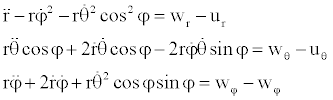(1)

where r is the relative distance between the missile and target; u and f are the aspect angles (Figure 1); wr, wΘ and wF are the target’s acceleration components; ur, uΘ and uF are the missile’s acceleration components, that are to be found, to the actuators with a saturation constraint that is defined as follows: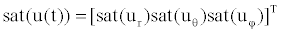(2)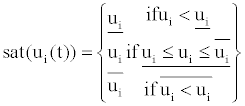(3)

For i=r,Θ and f

The kinematics (1) with input saturation constraint can be rewritten as the following nonlinear state-space equation .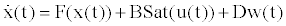(4)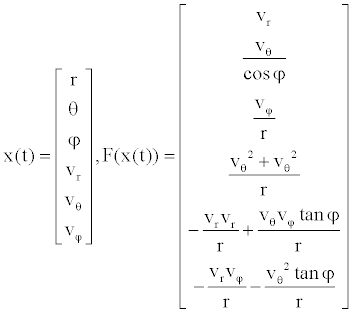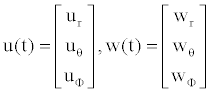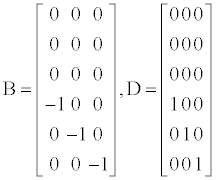Robust guidance law under unpredictable target acceleration w=0

We now prove that the proposed tunable guidance law can robustly stabilize the nonlinear missile-target dynamics (4) whether target acceleration w exists or not. In this section, we discuss the case of nontarget acceleration, w=0.

A good guidance law must guarantee a decreasing relative distance r and, at the same time, keep the LOS aspect angular rate as small as possible. It is equivalent to satisfying the following conditions:

Vr- radial relative velocity converges to negative value (5)

VΘ and VF- tangential relative velocities, converge to zero (6)

When VΘ and VF converge to zero, it means that the missile and target are on a convergence course. Only when the radial relative velocity, Vr, has decreased to a negative value, can the relative distance r between the missile and target decrease to zero.

Moon et al.  designed the guidance law for a two-dimensional air-to-air engagement whose state-space form is shown as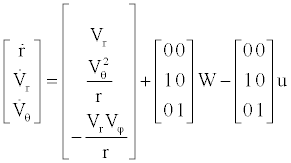(7)

They used variable structure control to design the control u in the form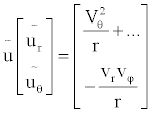(8)

From the control law (8) handling two-dimensional dynamics (7), it is reasonable to extend the form similar to (8) for the 3-D dynamics (4). Thus, the completely new control law, a form similar to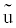in (8), is constructed mixed with some tunable parameters as follows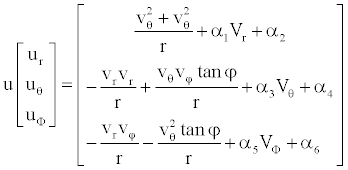(9)

Where ai, i = 1 - 6, are positive real constants. The saturation constraint of the actuators is given by (3). Suppose that the controller (9) lies between the upper and lower bound of saturation constraint (3). Substituting (9) into (4) yields the closed-loop system as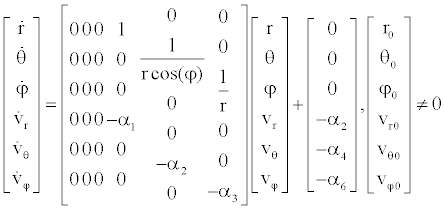(10)

Now consider a Lyapunov function candidate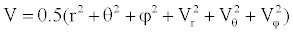for (10). Differentiating V with respect to time gives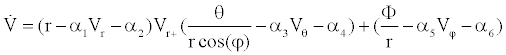(11)

In order to satisfy Lyapunov stability for (11),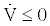Next, the parameters (ai, i=1-6) in (9) are considered. Rewriting the dynamics in (10)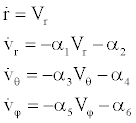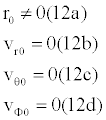(12)

where the solutions are shown as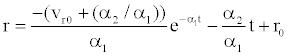(13a)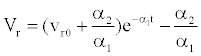(13b)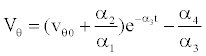(13c)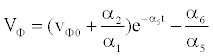(13d)

To tune the positive real numbers (a1, a3, a5) is equivalent to adjusting the convergent rate of (Vr, VΘ, Vf) in (13). The tunable parameters (a1, a3, a5) affect the final convergent state (Vr, VΘ, Vf) in (13). From (12b), it is known that the larger parameter a1 may cause quicker stabilization of Vr and the larger a2 may cause Vr to converge to a more negative value. From (12a), it is obvious that a more negative Vr would accelerate the convergence of the relative distance, r, between the missile and target, i.e., a good performance (less intercept time) for a non-accelerating target, w=0, is achieved. This is a completely new and original 3-D guidance design for tuning (a1, a2) so as to obtain less interception time and to tune (a3, a4, a5, a6) to handle the convergence of states in (10).

Parameter a1 is concerned with the convergence rate of Vr in (13b). A larger a2 may cause Vr to converge to a more negative value. a3 >0, a5>0 and a4=a6=0 in (12) guarantees that VΘ and Vf decrease to zero. In other words, the guidance law (9) with a1>0, a2>0 a3>0, a5>0 and a4=a6=0 can simultaneously satisfy the good guidance demand (5) and (6). Furthermore, the abrupt and large guidance command during the initial maneuver can also be avoided by tuning the four parameters (a1, a2, a3, a4). Thus, the proposed control law becomes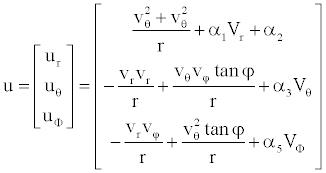(14)

The parameters (a, i=1, 2, 3, 5) of the control law are tuned by the binary search. This is an off-line tuning algorithm. If the performance demand is acceptable, the parameter’s selection is complete. There are many search algorithms that can be used to execute the tuning algorithm, for example, the genetic algorithm, binary search algorithm, and evolution algorithm. This paper adopts the binary search algorithm.

Robust guidance law under unpredictable target acceleration, w ≠ 0

Section 4.1 has shown that the proposed guidance law (9) can stabilize the states (r, Θ , f, Vr, VΘ, Vf) in the nonlinear missile - target dynamics (4) with non-target acceleration (w=0).

This section proves in detail that the proposed guidance law (14) can also resist the variations of the target’s maneuvers after adding the unpredictable target acceleration w to (4). Because the control law (14) satisfies the Lyapunov stability. Substituting the control law (14) into (4) yields the closed-loop system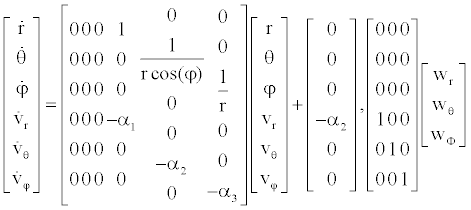(15)

The state equations including target acceleration w in (24) are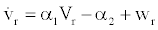(16)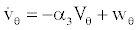(17)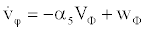(18)

where the target accelerations wr, wΘ and wF are regarded as disturbances. The robust guidance design objective can bestated as: to find the missile acceleration u=[ur, uΘ, uF]T , such that the L2 gains of the system states described by(16) - (18) are lower or equal to γ, the prescribed norm bound. That is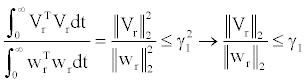(19)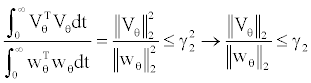(20)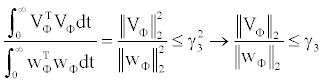(21)

where γ1, γ2 and γ3, are prescribed values that denote the effects of unpredictable acceleration components wr, wΘ and wF on Vr, VΘ and VF respectively. The following results provide sufficient conditions to satisfy robustness performance bound (19) - (21).

Theorem: Given (25),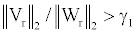holds if the following inequalities are satisfied.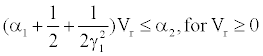(22a)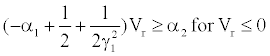(22b)

Sliding Mode Control (SMC)

The Sliding mode control approach is recognized as one of the efficient tool to design robust controllers for complex high-order nonlinear dynamic plant operating under uncertainty conditions.

The phenomenon “Sliding mode” may appear in dynamic systems governed by ordinary differential equations with discontinuous state functions. The conventional example of sliding mode-a second order relay system can be found in any text book on nonlinear control. The control input in the second order system.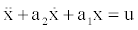alt="image"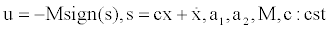may take only two values, M and -M, and undergoes discontinuities on the straight line s = 0 in the state plane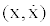. It follows from the analysis of the state plane that, in the neighborhood segment mn on the switching line sliding mode along this line. The equation of this line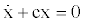may be interpreted as the sliding mode equation.

Sliding mode becomes the principle operation mode in so-called variable structures systems. A variable structure system consists of a set of continuous subsystems with a proper switching logic and, as a result, control actions are discontinuous functions of the system state, disturbances (if they are accessible for measurement), and reference inputs. The previous example of the relay system with state dependent amplitude of the control variable may serve as an illustration of a variable structure system: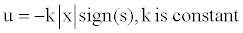Now the system with a1=0 and a2<0 consists of two unstable linear structures (u=kx and u=-kx) and u=-kx with x=0 and s=0 as switching lines. As it is clear from the system state plane, the state reaches the switching line s=0 for any initial conditions. Then, the sliding mode occurs on this line (Figure 2) with the motion equation, while the state vector decays exponentially.

Similarly, to the relay system, after the start of the sliding mode, the motion is governed by a reduced order equation which does not depend on the plant parameters.

Now we demonstrate sliding modes in non-linear affine systems of general from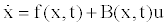(23)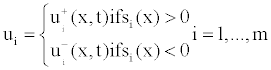(24)

Where xεRm is a state vector, uεRm is a control vector,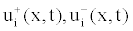and ( ) i S x are continuous functions of their arguments,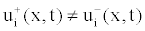. The control is discontinuities in some surface in the system state space.

Sliding mode control problem

Consider the problem of doing set point control for a system of the form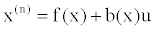Further, we seek a solution that is robust to uncertainties in f(x) and b(x). Note that the system can be rewritten in vector form. It is then equivalent to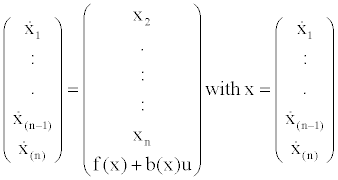Define xd (the set-point) and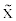(the error signal) the difference between x and xd.

We take a 2 step approach to design the controller:

1. Define the sliding mode: This is a surface that is invariant of the controlled dynamics, where the controlled dynamics are exponentially stable, and where the system tracks the desired set-point.

2. Define the control that drives the state to the sliding mode in finite time.

Define the sliding mode s(t) as follows: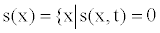(25)

where s(x,t) is defined by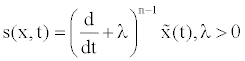(26)

Note that on the surface s(t) the error dynamics are governed by the equation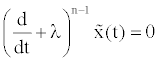(27)

On this surface the error will converge to 0 exponentially.

This implies that if there exists a control input u(t) such that x(t) is in s(t) it follows that x(t) is in s(t) for all T>t and the error will converge exponentially to 0 for this control input.

Remarks

The choice of s(x,t) is somewhat arbitrary. You may choose any error dynamic which leads to exponentially stable behavior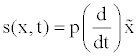(28)

where p(s) is a polynomial with all zeros in the left half plane, then the error dynamics will converge exponentially.

The strategy to converge to the sliding mode is that we add something to u(t) which will drive us to the sliding mode in finite time.

More formally, we assume that for all x,b(x) ≠ 0 . To prove that the sliding mode exists, we derive a control such that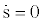. With s(x,t) defined as above: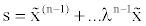Differentiating: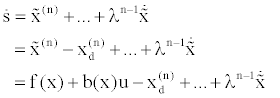(30)

Defining u as: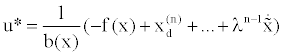Then for u *= u it is clear that for all time. In order to complete the second control design step, we consider the following control: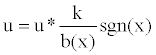(31)

where: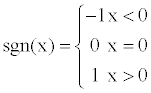To prove convergence to the sliding mode, we show that with this control, s will converge to 0 in finite time. Consider the development of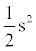over time. Differentiating gives: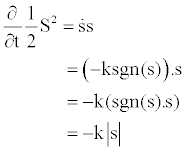Thus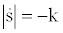, so we get convergence to the sliding mode in finite time.

In summary, the motion consists of a reaching phase during in which trajectories starting off the manifold s=0 move toward it and reach it in finite time, followed by a sliding phase during which the motion is confined to the manifold s=0 and the dynamics of the system are represented by a reduced order model with exponentially stable error dynamics. The manifold s=0 is called the sliding mode, and the control law

u = -ß (x)sgn(s) is called sliding control mode.

Sliding mode for ur, uΘ and uF (where are the missile’s acceleration components) shown in Figure 3.

Simulation example

In this section, a numerical simulation justifies the availability and superiority of the proposed method in some scenario.

One maneuvering strategy of targets in 3-D is employed to examine the robustness and tracking performance of the guidance law; that is, the external disturbance w(t) in (4) is generated by the following maneuvering step target to test the robustness in this example.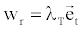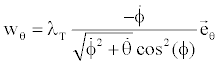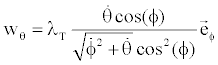Where λT is the target’s navigation gain with random value within 0 - 4 G and O = 20 rad/s. This scenario is proposed to demonstrate the performance robustness of the proposed method.

Let Target escape from missile (wr> 0)

r = 4Km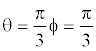Vr = -500m/s, VΘ = 200m/s, VF= 300m/s

Table 1 shows time and distance both as controllers. Also, it represents the characteristics of each method. In Figure 4 two guidance laws are compared to each other. From the view point of short interceptive time, the proposed guidance is more successful than that of Chen et al.  and Shieh . It should be emphasized that the target models are only for demonstrating the feasibility of the proposed method. Finally, Figure 5 shows variables and their changes along time.

Time &distance Controller
H Sliding mode
Interception Time (s) 6.74 6.09
Mass Distance r (meter) 0.46 0.65

Table 1: Characteristics controller proposed.

Figure 4: Trajectories of relative missile-target distance r and missile acceleration with Coefficients H∞ :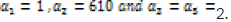#### Conclusion

In this paper, the three-dimensional kinematics equations which describe the moving relationships of the missile and target are established.

A completely new robust guidance law with a saturation constraint against target escape is proposed. By regarding the target maneuvers as unpredictable disturbance inputs, the missile-target nonlinear 3-D guidance problems considered as a nonlinear disturbance attenuation control problem. Without solving the complicated Hamilton-Jacobi Partial Differential Inequality (HJPDI), a guidance law is proposed to achieve the design goal with less interruption time.

Finally, some sufficient condition is addressed supporting our guidance law having H and sliding mode robust performance. An illustrative example has been proposed to demonstrate that the interception time was less than that proposed by other guidance laws.

#### References

Select your language of interest to view the total content in your interested language

### Article Usage

• Total views: 13430
• [From(publication date):
July-2013 - Jan 24, 2020]
• Breakdown by view type
• HTML page views : 9505Can't read the image? click here to refresh